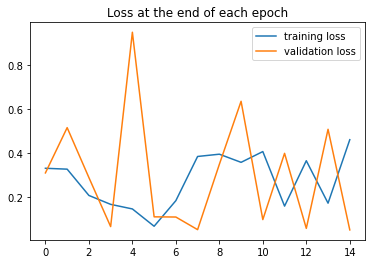# Loss won't decrease

Hi all,

I’m new to this forum and PyTorch in general. I’ve been trying to train a CNN model to do a binary image classification task. The accuracy results seem good but the loss is all over the place and I have no idea what I’m doing wrong.

I have 68538 training images and 32809 testing images available.

Any advice/suggestions would be much appreciated.``````import torch
import torch.nn as nn
import torch.nn.functional as F
import torch.optim as optim
from torchvision import datasets, transforms, models
from torchvision.utils import make_grid
import os
import copy

import numpy as np
import pandas as pd
import matplotlib.pyplot as plt
%matplotlib inline
``````
``````device = torch.device("cuda" if torch.cuda.is_available() else "cpu")
if torch.cuda.device_count() > 0:
print("Running on : ", torch.cuda.device_count(), " GPUs!"
if torch.cuda.device_count() > 1 else " GPU!")
else:
print("Running on CPU!")
``````
``````train_transform = transforms.Compose([
transforms.RandomRotation(359),
transforms.RandomHorizontalFlip(),
transforms.RandomVerticalFlip(),
transforms.Resize(224),
transforms.CenterCrop(224),
transforms.ToTensor(),
transforms.Normalize([0.485, 0.456, 0.406],
[0.229, 0.224, 0.225])
])

test_transform = transforms.Compose([
transforms.Resize(224),
transforms.CenterCrop(224),
transforms.ToTensor(),
transforms.Normalize([0.485, 0.456, 0.406],
[0.229, 0.224, 0.225])
])
``````
``````root = 'C:/Concrete2'

train_data = datasets.ImageFolder(os.path.join(root, 'train'), transform=train_transform)
test_data = datasets.ImageFolder(os.path.join(root, 'test'), transform=test_transform)

torch.manual_seed(283)
``````
``````class ConvolutionalNetwork(nn.Module):
def __init__(self):
super().__init__()
self.conv1 = nn.Conv2d(3, 6, 3, 1)
self.conv2 = nn.Conv2d(6, 16, 3, 1)
self.fc1 = nn.Linear(54*54*16, 120)
self.fc2 = nn.Linear(120, 84)
self.fc3 = nn.Linear(84, 2)

def forward(self, X):
X = F.relu(self.conv1(X))
X = F.max_pool2d(X, 2, 2)
X = F.relu(self.conv2(X))
X = F.max_pool2d(X, 2, 2)
X = X.view(-1, 54*54*16)
X = F.relu(self.fc1(X))
X = F.relu(self.fc2(X))
X = self.fc3(X)
return F.log_softmax(X, dim=1)
``````
``````torch.manual_seed(462)
CNNmodel = ConvolutionalNetwork().to(device)
criterion = nn.CrossEntropyLoss()
``````
``````import time
start_time = time.time()

epochs = 15

train_losses = []
test_losses = []
train_correct = []
test_correct = []

for i in range(epochs):
trn_corr = 0
tst_corr = 0

# Run the training batches
for b, (X_train, y_train) in enumerate(train_loader):
X_train = torch.FloatTensor(X_train).to(device)
y_train = torch.LongTensor(y_train).to(device)

b+=1

# Apply the model
y_pred = CNNmodel(X_train)
loss = criterion(y_pred, y_train)

# Tally the number of correct predictions
predicted = torch.max(y_pred.data, 1)
batch_corr = (predicted == y_train).sum()
trn_corr += batch_corr

# Update parameters
loss.backward()
optimizer.step()

# Print interim results
if b%457 == 0:
print(f'epoch: {i:2}  batch: {b:4} [{50*b:6}/68538]  loss: {loss.item():10.8f}  \
accuracy: {trn_corr.item()*100/(50*b):7.3f}%')

train_losses.append(loss)
train_correct.append(trn_corr)

# Run the testing batches
for b, (X_test, y_test) in enumerate(test_loader):
X_test = torch.FloatTensor(X_test).to(device)
y_test = torch.LongTensor(y_test).to(device)

# Apply the model
y_val = CNNmodel(X_test)

# Tally the number of correct predictions
predicted = torch.max(y_val.data, 1)
tst_corr += (predicted == y_test).sum()

loss = criterion(y_val, y_test)
test_losses.append(loss)
test_correct.append(tst_corr)

print(f'\nDuration: {time.time() - start_time:.0f} seconds') # print the time elapsed
``````

few things can you place ` optimizer.zero_grad()` just after the for loop `for b, (X_train, y_train) in enumerate(train_loader):` and secondly your training learning rate is pretty low. try increasing the learning rate to see if anything changes`nn.CrossEntropyLoss` expects raw logits as the model outputs so you should either remove the `F.log_softmax` usage in your model or use `nn.NLLLoss` alternatively with `F.log_softmax`.
If you are using `nn.CrossEntropyLoss`, you should remove it completely. It wouldn’t change the result, since `F.log_softmax(x, dim=1) == F.log_softmax(F.log_softmax(x, dim=1), dim=1)`, but it’s unnecessary.